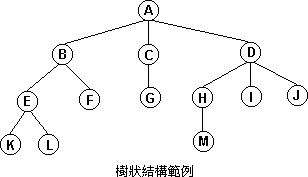# [C++程式設計] 學習筆記─建立資料結構(2/5)1.有一個特定的節點稱為根節點 ( root )。
2.其餘的節點分成 n >= 0 個獨立的集合 T1 , T2,…..,Tn , 其中的各個集合是一個樹狀結構。即稱T1 , T2,　　　　…..,Tn 為根節點的子樹 ( Subtree ) 。※建立所需的資料結構

`   1:  class Vertex{`
`   2:           char * V_name;`
`   3:           int V_type;`
`   4:           int V_area;`
`   5:           int V_power;`
`   6:           int V_layer;`
`   7:  };`

`   1:  int a;`

`   1:  int b;`

 b b b b b b b b b b

`   1:  Vertex VertexID;`

Vertex VertexID;

 VertexID {V_name;V_type;V_area;V_power;V_layer;} VertexID VertexID VertexID VertexID

 0 1 2 3 4 V_name V_name V_name V_name V_name V_type V_type V_type V_type V_type V_area V_area V_area V_area V_area V_power V_power V_power V_power V_power V_layer V_layer V_layer V_layer V_layer

VertexID.V_name = ‘R’;

Copyright Rami Reserved.

### About the author